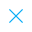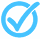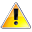Call Support +91-85588-96644

Keep me logged in
You can't leave Captcha Code empty
By submitting this form, you agree to the Terms & Privacy Policy.
ORTests given

OR# Statistics - II

Questions

Time

Highest
score

Level

## ENGLISH

Language

Topics Covered:StatisticsFormula for Calculating Median in Grouped DataFormula for Calculating Median in Grouped DataFormula for Calculating Median in Grouped DataFormula for Calculating Median in Grouped DataFormula for Calculating Median in Grouped DataFormula for Calculating Median in Grouped DataFormula for Calculating Median in Grouped DataFormula for Calculating Median in Grouped DataFormula for Calculating Median in Grouped DataThis is a concept-building practice test and may not have exact structure as you would expect in the actual exam. Please exercise your discretion to attempt it or go to structured Featured Section.

Description:
Statistics - II
Keywords:
 Type Comment Question
Topper(s) of this Test
•### Rashika

Score: 40/40

•### Ayush Jain

Score: 39/40

•### Anubhav Jain

Score: 39/40

•### Dhruv Dutta

Score: 39/40

•### Gourav Sandhu

Score: 38/40Related Tests